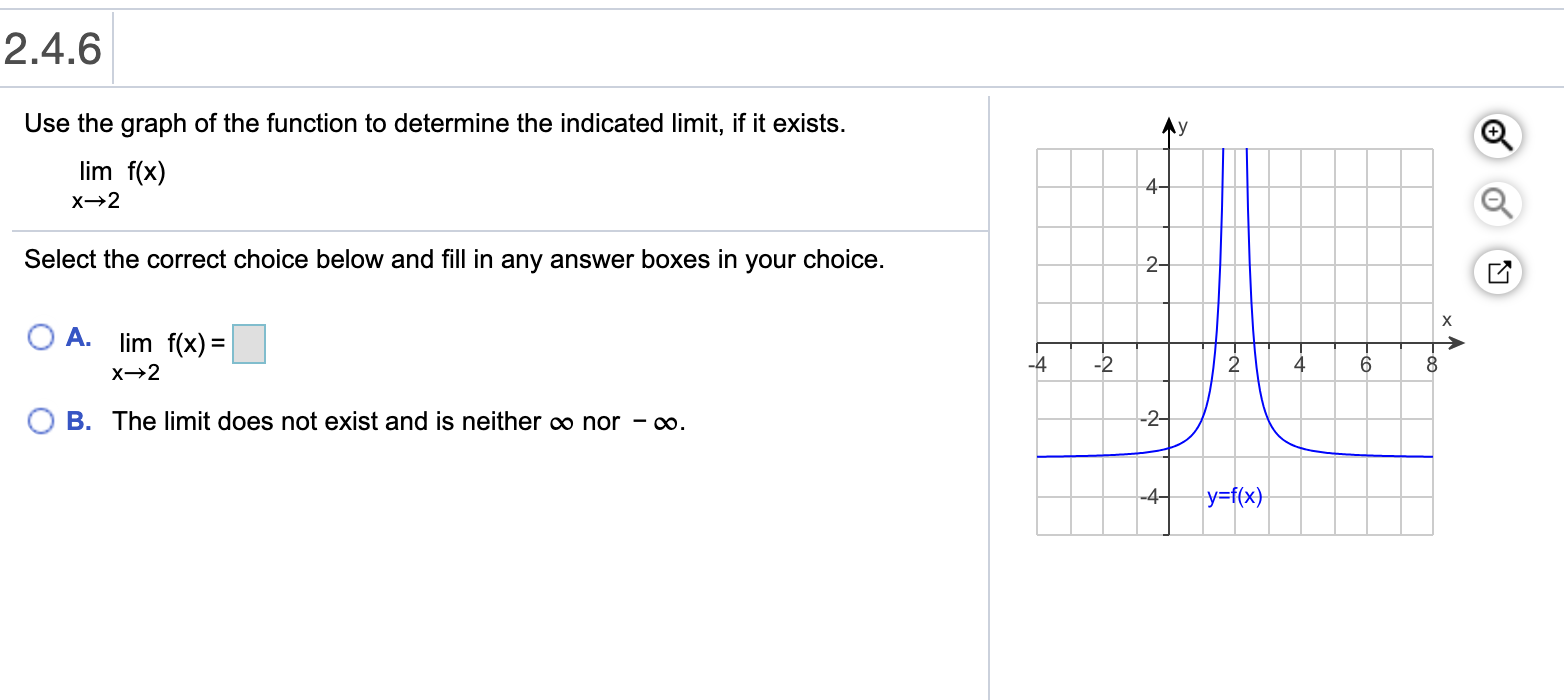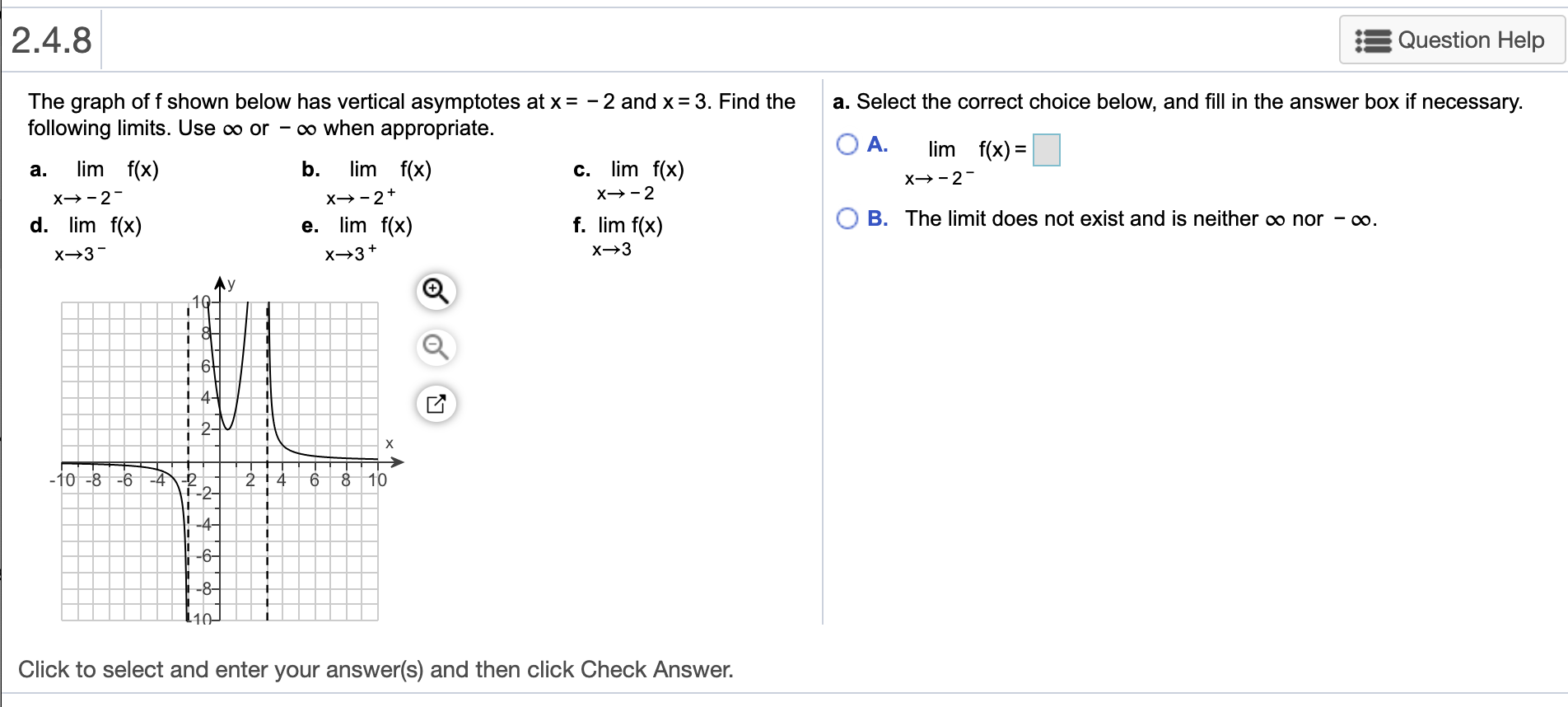# 2.4.6Use the graph of the function to determine the indicated limit, if it exists.Aylim f(x)4-Select the correct choice below and fill in any answer boxes in your choice.2-O A. lim f(x) =|-4-24B. The limit does not exist and is neither o nor - o.-2-y=f(x}-4-of 2.4.8Question HelpThe graph off shown below has vertical asymptotes at x = - 2 and x= 3. Find thefollowing limits. Use o or - o when appropriate.a. Select the correct choice below, and fill in the answer box if necessary.O A.lim f(x) =lim f(x)lim f(x)b.limf(x)a.C.x→-2-X→-2X→-2+x→-2-B. The limit does not exist and is neither o nor - ∞.f. lim f(x)d. lim f(x)e. lim f(x)х—3-X→3+Ay+ 6t2-х-4F-2-2 148.-10 -8 -610|4-%3D-6--8-140Click to select and enter your answer(s) and then click Check Answer.

Question
6 viewshelp_outlineImage Transcriptionclose2.4.6 Use the graph of the function to determine the indicated limit, if it exists. Ay lim f(x) 4- Select the correct choice below and fill in any answer boxes in your choice. 2- O A. lim f(x) =| -4 -2 4 B. The limit does not exist and is neither o nor - o. -2- y=f(x} -4- of fullscreenhelp_outlineImage Transcriptionclose2.4.8 Question Help The graph off shown below has vertical asymptotes at x = - 2 and x= 3. Find the following limits. Use o or - o when appropriate. a. Select the correct choice below, and fill in the answer box if necessary. O A. lim f(x) = lim f(x) lim f(x) b. lim f(x) a. C. x→-2- X→-2 X→-2+ x→-2- B. The limit does not exist and is neither o nor - ∞. f. lim f(x) d. lim f(x) e. lim f(x) х—3- X→3+ Ay + 6t 2- х -4 F-2- 2 14 8. -10 -8 -6 10 |4- %3D -6- -8- 140 Click to select and enter your answer(s) and then click Check Answer. fullscreen
check_circle

Step 1

To find: -

Step 2

Calculation: -

Step 3

Now,

...

### Want to see the full answer?

See Solution

#### Want to see this answer and more?

Solutions are written by subject experts who are available 24/7. Questions are typically answered within 1 hour.*

See Solution
*Response times may vary by subject and question.
Tagged in

### Functions